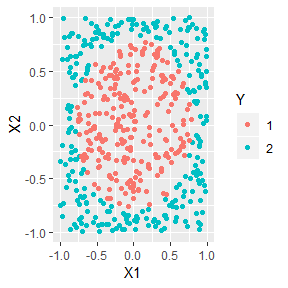# Package Introduction

DANN is a variation of k nearest neighbors where the shape of the neighborhood takes into account training data’s class. The neighborhood is elongated along class boundaries and shrunk in the orthogonal direction to class boundaries. See Discriminate Adaptive Nearest Neighbor Classification by Hastie and Tibshirani. This package implements DANN and sub-DANN in section 4.1 of the publication and is based on Christopher Jenness’s python implementation.

# Arguments

• k - The number of points in the neighborhood. Identical to k in standard k nearest neighbors.
• neighborhood_size - The number of data points used to estimate class boundaries.
• epsilon - Softening parameter. Usually has the least affect on performance.

# Example: Circle Data

In what follows a train and test set are created. Class 1 is inside a circle and class 2 surrounds class 1. dann is an accurate model for these data.

library(dann)
library(mlbench)
library(magrittr)
library(dplyr, warn.conflicts = FALSE)
library(ggplot2)

######################
# Circle Data
######################
set.seed(1)
train <- mlbench.circle(500, 2) %>%
tibble::as_tibble()
colnames(train) <- c("X1", "X2", "Y")

ggplot(train, aes(x = X1, y = X2, colour = Y)) +
geom_point()xTrain <- train %>%
select(X1, X2) %>%
as.matrix()

yTrain <- train %>%
pull(Y) %>%
as.numeric() %>%
as.vector()

test <- mlbench.circle(500, 2) %>%
tibble::as_tibble()
colnames(test) <- c("X1", "X2", "Y")

xTest <- test %>%
select(X1, X2) %>%
as.matrix()

yTest <- test %>%
pull(Y) %>%
as.numeric() %>%
as.vector()

dannPreds <- dann(xTrain, yTrain, xTest, 3, 50, 1, FALSE)
mean(dannPreds == yTest) #An accurate model.
#>  0.96

rm(train, test)
rm(xTrain, yTrain)
rm(xTest, yTest)
rm(dannPreds)